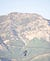# Functional JavaScript — The BasicsPhoto by Tijana Drndarski on Unsplash

JavaScript is partly a functional language.

To learn JavaScript, we got to learn the functional parts of JavaScript.

In this article, we’ll look at how to use the functional programming features in JavaScript.

# What Is Functional Programming?

Functional programming is the use of various principles in our app’s code.

The most basic principle is that we abstract out the how part into reusable code.

And we create functions that don’t depend on the outside.

Also, if the function have the same input, we get the same output.

Functions are mathematical functions.

They take arguments and return values.

The arguments we pass in will always create the same output if the arguments are the same.

Also, they don’t interact with the outside world.

For instance, we can have a function like:

`const calculateTax = (value, percentValue) => {  return value / 100 * (100 +    percentValue)}`

It takes some arguments and doesn’t reference anything outside in the function body.

This is called a pure function.

A pure function is a function that doesn’t reference anything outside.

And if we give it the same inputs, it’ll always give the same outputs.

# JavaScript Functions vs Methods

A function is a piece of code that can be called by its name.

It can pass arguments and return values.

A method is a piece of code that may be called by its name with its associated object name.

So a function is something like:

`const foo = (a) => {  return a}`

And a method is something like:

`const obj = {  foo: (a) => {    return a  }}`

We call the method by writing:

`obj.foo(1)`

# Referential Transparency

Functions return the same output for the same input.

This property is called referential transparency.

If we have referential transparent, then we can infer the return value from the function call.

So if we have:

`const sameVal = (i) => {  return i}`

and:

`sum(4,5) + sameVal(1)`

We can infer that the expression above is the same as:

`sum(4,5) + 1`

The substitution model lets us substitute the direct result of a function.

This leads to parallel code and caching since they don’t depend on anything outside.

All they depend on is their argument.

# Imperative, Declarative, Abstraction

Functional programming is about being declarative and writing abstracted code.

Declarative code means we tell the computer what the compiler needs to do rather than how to do it.

The how part is abstracted into higher-order functions.

Higher-order functions are fuincti8ons that take functions as arguments or return functions.

For example, the array instance `forEach` method takes a callback and then log an element.

We can iterate through an array with it by writing:

`const array = [1, 2, 3];array.forEach((a) => console.log(a))`Photo by Maxwell Nelson on Unsplash

# Conclusion

Functional programming follows some basic principles.

We create functions that don’t depend on the outside and if we give them the same inputs, we always get the same outputs.

Web developer. Subscribe to my email list now at https://thewebdev.info/subscribe/. Email me at hohanga@gmail.com

Web developer. Subscribe to my email list now at https://thewebdev.info/subscribe/. Email me at hohanga@gmail.com